# Chapter 4 - Under the Hood - Training a Digit Classifier¶

Deep Learning For Coders with fastai & Pytorch - Under the Hood - Training a Digit Classifier. In this notebook. I add some cells for utility fuctions. path, ls, untar, !, tree usage, as usual I followed both Jeremy Howard's Lesson and Weights and Biases reading group videos. Click open in colab button at the right side to view as notebook.

• toc: true
• categories: [fastbook]
• image: images/magpie.pngI found this little one in front of my window. Suffering from foot deformity and can't fly. Now fully recovered and back his/her family.

In :
#!pip install -Uqq fastbook
import fastbook
fastbook.setup_book()
# below is for disabling Jedi autocomplete that doesn't work well.
%config Completer.use_jedi = False

In :
from fastai.vision.all import *
from fastbook import *

matplotlib.rc('image', cmap='Greys')


## EXPLORING THE DATASET¶

### What untar does?¶

Note: 'untar_data' come from fastai library, it downloads the data and untar it if it didn't already and returns the destination folder.

In :
path = untar_data(URLs.MNIST_SAMPLE)

In :
??untar_data


Tip: Check it with '??'

### What is path ?¶

In :
path

Out:
Path('.')

Tip: what is inside the current folder? this where the jupyter notebook works. '!' at the beginning means the command works on the terminal.

### What is !ls ?¶

Note: ls works on the terminal. (-d for only listing directories)

In :
!ls

2020-02-20-test.ipynb	    ghtop_images  my_icons


can be used like this too.

In :
!ls /home/niyazi/.fastai/data/mnist_sample/train -d

/home/niyazi/.fastai/data/mnist_sample/train


also like this:

In :
!ls /home/niyazi/.fastai/data/mnist_sample/train/3 -d

/home/niyazi/.fastai/data/mnist_sample/train/3


### What is tree ?¶

Note: for seeing tree sturucture of the files and folders (-d argument for directories)

In :
!tree /home/niyazi/.fastai/data/mnist_sample/ -d

/home/niyazi/.fastai/data/mnist_sample/
├── train
│   ├── 3
│   └── 7
└── valid
├── 3
└── 7

6 directories

In :
Path.BASE_PATH = path


### What is ls() ?¶

Note: 'ls' is method by fastai similiar the Python's list fuction but more powerful.

In :
path.ls()

Out:
(#3) [Path('labels.csv'),Path('train'),Path('valid')]

Note: Check this usage:

In :
(path/'train')

Out:
Path('train')
In :
(path/'train').ls()

Out:
(#2) [Path('train/7'),Path('train/3')]

Note: there are two folders under training folder

In :
threes = (path/'train'/'3').ls().sorted()
sevens = (path/'train'/'7').ls().sorted()


Note: this code returns and ordered list of paths

### What is PIL ? (Python Image Library)¶

In :
im3_path = threes
im3 = Image.open(im3_path)
type(im3)
#im3

Out:
PIL.PngImagePlugin.PngImageFile

### NumPy array¶

The 4:10 indicates we requested the rows from index 4 (included) to 10 (not included) and the same for the columns. NumPy indexes from top to bottom and left to right, so this section is located in the top-left corner of the image.

In :
array(im3)[4:10,4:10]

Out:
array([[  0,   0,   0,   0,   0,   0],
[  0,   0,   0,   0,   0,  29],
[  0,   0,   0,  48, 166, 224],
[  0,  93, 244, 249, 253, 187],
[  0, 107, 253, 253, 230,  48],
[  0,   3,  20,  20,  15,   0]], dtype=uint8)

Note: this is how it looks some part of the image in the NumPy array

### Pytorch tensor¶

Here's the same thing as a PyTorch tensor:

In :
tensor(im3)[4:10,4:10]

Out:
tensor([[  0,   0,   0,   0,   0,   0],
[  0,   0,   0,   0,   0,  29],
[  0,   0,   0,  48, 166, 224],
[  0,  93, 244, 249, 253, 187],
[  0, 107, 253, 253, 230,  48],
[  0,   3,  20,  20,  15,   0]], dtype=torch.uint8)

Note: It is possible to convert it to a tansor as well.

In :
im3_t = tensor(im3)
df = pd.DataFrame(im3_t[4:15,4:22])

Out:
0 1 2 3 4 5 6 7 8 9 10 11 12 13 14 15 16 17
0 0 0 0 0 0 0 0 0 0 0 0 0 0 0 0 0 0 0
1 0 0 0 0 0 29 150 195 254 255 254 176 193 150 96 0 0 0
2 0 0 0 48 166 224 253 253 234 196 253 253 253 253 233 0 0 0
3 0 93 244 249 253 187 46 10 8 4 10 194 253 253 233 0 0 0
4 0 107 253 253 230 48 0 0 0 0 0 192 253 253 156 0 0 0
5 0 3 20 20 15 0 0 0 0 0 43 224 253 245 74 0 0 0
6 0 0 0 0 0 0 0 0 0 0 249 253 245 126 0 0 0 0
7 0 0 0 0 0 0 0 14 101 223 253 248 124 0 0 0 0 0
8 0 0 0 0 0 11 166 239 253 253 253 187 30 0 0 0 0 0
9 0 0 0 0 0 16 248 250 253 253 253 253 232 213 111 2 0 0
10 0 0 0 0 0 0 0 43 98 98 208 253 253 253 253 187 22 0

## BASELINE: Pixel similarity¶

In :
seven_tensors = [tensor(Image.open(o)) for o in sevens]
three_tensors = [tensor(Image.open(o)) for o in threes]
len(three_tensors),len(seven_tensors)

Out:
(6131, 6265)

Note: 'sevens' are still list of paths. 'o' is a path in the list, then with the list comprehension we use the path to read the image, then cast the image into tensor.(Same for threes). 'seven_tensor' is a list of tensors

In :
show_image(three_tensors);Tip: Show image shows the first tensor as image

In :
show_image(tensor(im3))

Out:
<AxesSubplot:>Note: check this in more straight way (im3>tensor>image)

### Training Set: Stacking Tensors¶

In :
stacked_sevens = torch.stack(seven_tensors).float()/255
stacked_threes = torch.stack(three_tensors).float()/255
stacked_threes.shape

Out:
torch.Size([6131, 28, 28])
In :
type(stacked_sevens)

Out:
torch.Tensor
In :
type(stacked_sevens)

Out:
torch.Tensor
In :
type(seven_tensors)

Out:
list

Note: now we turn our list into a tensor size of ([6131, 28, 28])

In :
len(stacked_threes.shape)

Out:
3

Note: This is rank (lenght of the shape)

In :
stacked_threes.ndim

Out:
3

Note: This is more direct way to get it. (ndim)

### Mean of threes and sevens our ideal 3 and 7.¶

In :
mean3 = stacked_threes.mean(0)
show_image(mean3);Note: This is the mean of the all tensors through first axis. 'Ideal Three'

In :
mean7 = stacked_sevens.mean(0)
show_image(mean7);In :
a_3 = stacked_threes
show_image(a_3);### Distance between the ideal three and other threes¶

In :
dist_3_abs = (a_3 - mean3).abs().mean()
dist_3_sqr = ((a_3 - mean3)**2).mean().sqrt()
dist_3_abs,dist_3_sqr

Out:
(tensor(0.1114), tensor(0.2021))
In :
dist_7_abs = (a_3 - mean7).abs().mean()
dist_7_sqr = ((a_3 - mean7)**2).mean().sqrt()
dist_7_abs,dist_7_sqr

Out:
(tensor(0.1586), tensor(0.3021))

Note: Then we need to calculate the distance between the 'ideal' and ordinary three.Two methods for getting the distance L1 Norm and MSE second one is panelize bigger mistake more havil, L1 is uniform.

It is obvious that a_3 is closer to the perfect 3 so our approach worked at this time. (Both in L1 and MSE)

### Pytorch L1 and MSE fuctions¶

In :
F.l1_loss(a_3.float(),mean7), F.mse_loss(a_3,mean7).sqrt()

Out:
(tensor(0.1586), tensor(0.3021))

Note: torch.nn.functional as F (for mse, manually take the sqrt)

Important: (from notebook) If you don't know what C is, don't worry as you won't need it at all. In a nutshell, it's a low-level (low-level means more similar to the language that computers use internally) language that is very fast compared to Python. To take advantage of its speed while programming in Python, try to avoid as much as possible writing loops, and replace them by commands that work directly on arrays or tensors.

### Array and Tensor Examples¶

In :
data = [[1,2,3],[4,5,6]]
arr = array (data)
tns = tensor(data)

In :
arr  # numpy

Out:
array([[1, 2, 3],
[4, 5, 6]])
In :
tns  # pytorch

Out:
tensor([[1, 2, 3],
[4, 5, 6]])

In :
tns[:,1]

Out:
tensor([2, 5])
In :
tns[1,1:3]

Out:
tensor([5, 6])
In :
tns+1

Out:
tensor([[2, 3, 4],
[5, 6, 7]])
In :
tns.type()

Out:
'torch.LongTensor'
In :
tns*1.5

Out:
tensor([[1.5000, 3.0000, 4.5000],
[6.0000, 7.5000, 9.0000]])

### Validation set :Stacking Tensors¶

In :
valid_3_tens = torch.stack([tensor(Image.open(o))
for o in (path/'valid'/'3').ls()])
valid_3_tens = valid_3_tens.float()/255
valid_7_tens = torch.stack([tensor(Image.open(o))
for o in (path/'valid'/'7').ls()])
valid_7_tens = valid_7_tens.float()/255
valid_3_tens.shape,valid_7_tens.shape

Out:
(torch.Size([1010, 28, 28]), torch.Size([1028, 28, 28]))

### Manual L1 distance function¶

In :
def mnist_distance(a,b): return (a-b).abs().mean((-1,-2))
mnist_distance(a_3, mean3)

Out:
tensor(0.1114)

In :
valid_3_dist = mnist_distance(valid_3_tens, mean3)
valid_3_dist, valid_3_dist.shape

Out:
(tensor([0.1270, 0.1254, 0.1114,  ..., 0.1494, 0.1097, 0.1365]),
torch.Size())

Note:I think this an example of not using loops which slows down the process (check above important tag). Although shapes of the tensors don't match, out function still works. Pytorch fills the gaps.

here is another example. Shapes don't match.

In :
tensor([1,2,3]) + tensor(1)

Out:
tensor([2, 3, 4])
In :
(valid_3_tens-mean3).shape

Out:
torch.Size([1010, 28, 28])
In :
def is_3(x): return mnist_distance(x,mean3) < mnist_distance(x,mean7)

In :
is_3(a_3), is_3(a_3).float()

Out:
(tensor(True), tensor(1.))

#### here is an another broadcasting for all validation set:¶

In :
is_3(valid_3_tens)

Out:
tensor([True, True, True,  ..., True, True, True])

### Accuracy of our 'ideal' 3 and 7¶

In :
accuracy_3s =      is_3(valid_3_tens).float() .mean()
accuracy_7s = (1 - is_3(valid_7_tens).float()).mean()

accuracy_3s,accuracy_7s,(accuracy_3s+accuracy_7s)/2

Out:
(tensor(0.9168), tensor(0.9854), tensor(0.9511))

Arthur Samues Machine Learning process:

• Initialize the weights.
• For each image, use these weights to predict whether it appears to be a 3 or a 7.
• Based on these predictions, calculate how good the model is (its loss).
• Calculate the gradient, which measures for each weight, how changing that weight would change the loss (SGD)
• Step (that is, change) all the weights based on that calculation.
• Go back to the step 2, and repeat the process.
• Iterate until you decide to stop the training process (for instance, because the model is good enough or you don't want to wait any longer).
In :
#id gradient_descent
#alt Graph showing the steps for Gradient Descent
gv('''
step->predict[label=repeat]
''')

Out:

### GD example¶

In :
def f(x): return x**2

In :
plot_function(f, 'x', 'x**2')
plt.scatter(-1.5, f(-1.5), color='red');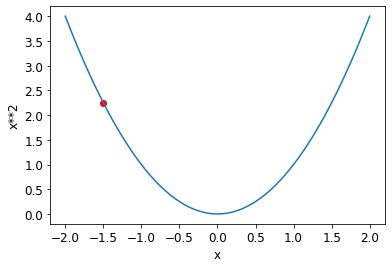We need to decrease the lossNow our tensor xt is under investigation. Pytorch will keeps its eye on it.

In :
xt = tensor(3.).requires_grad_()

In :
yt = f(xt)
yt

Out:
tensor(9., grad_fn=<PowBackward0>)

Result is 9 but there is a grad function in the result.

In :
yt.backward()


backward calculates the derivative.

In :
xt.grad

Out:
tensor(6.)

result is 6.

now with a bigger tensor

In :
xt = tensor([3.,4.,10.]).requires_grad_()
xt

Out:
tensor([ 3.,  4., 10.], requires_grad=True)
In :
def f(x): return (x**2).sum()

In :
yt = f(xt)
yt

Out:
tensor(125., grad_fn=<SumBackward0>)

again we expect 2*xt:

In :
yt.backward()

In :
xt.grad

Out:
tensor([ 6.,  8., 20.])

### End to end SGD example¶

In :
time = torch.arange(0,20).float()
time

Out:
tensor([ 0.,  1.,  2.,  3.,  4.,  5.,  6.,  7.,  8.,  9., 10., 11., 12., 13., 14., 15., 16., 17., 18., 19.])
In :
speed = torch.randn(20)*3 + 0.75*(time-9.5)**2 + 1
plt.scatter(time,speed)

Out:
<matplotlib.collections.PathCollection at 0x7f8f2edd27c0>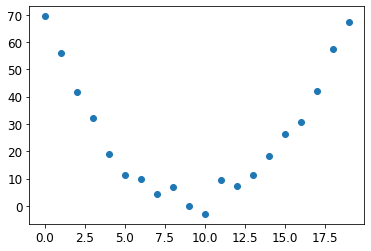Now we are trying to come up with some parameters for our quadratic fuction that predicts speed any given time. Our choice is quadratic but that could be something else too. with a quadratic function our problem would be much easier.

here is the function gets time and parameter as inputs and predicts a result:

In :
def f(t, params):
a,b,c = params
return a*(t**2) + (b*t) + c


this our loss function that calculate distance between prediction and target( actual mesurements)

In :
def mse(preds, targets): return ((preds-targets)**2).mean().sqrt()


#### Step 1: here are initial random parameters:¶

In :
params = torch.randn(3).requires_grad_()
params

Out:
tensor([ 0.9569,  0.0048, -0.1506], requires_grad=True)
In :
orig_params = params.clone()


#### Step 2: calculate predictions:¶

In :
preds = f(time,params)

In :
def show_preds(preds, ax=None):
if ax is None: ax=plt.subplots()
ax.scatter(time, speed)
ax.scatter(time, to_np(preds), color='red')
ax.set_ylim(-300,100)

In :
show_preds(preds)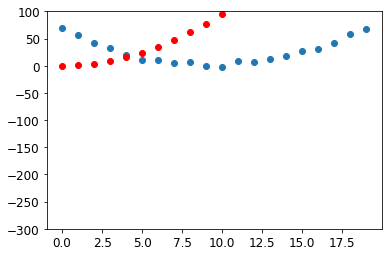#### Step 3: Calculate the loss¶

In :
loss = mse(preds,speed)
loss

Out:
tensor(139.3082, grad_fn=<SqrtBackward>)

The Question is how to improve these results:

#### Step 4: first we calculate the gradient:¶

Pytorch makes it easier we just call the backward() on the loss but it calculates gradient for the params 'a' 'b' and 'c'._

In :
loss.backward()
params.grad # this is the derivative of the initial values in other word our slope.

Out:
tensor([165.0324,  10.5991,   0.6615])
In :
params.grad * 1e-5 # scaler at the end is learning rate.

Out:
tensor([1.6503e-03, 1.0599e-04, 6.6150e-06])
In :
params # they are still same.

Out:
tensor([ 0.9569,  0.0048, -0.1506], requires_grad=True)

#### Step 5: Step the weight.¶

we picked the learning rate 1e-5 very small step to avoid missing the lowest possible loss.

In :
lr = 1e-5

In :
preds = f(time,params)
mse(preds, speed)

Out:
tensor(139.0348, grad_fn=<SqrtBackward>)

lets create a function for all these steps

In :
def apply_step(params, prn=True):
preds = f(time, params)
loss = mse(preds, speed)
loss.backward()
if prn: print(loss.item())
return preds


#### Step 6: repeat the step:¶

In :
for i in range(10): apply_step(params)

139.03475952148438
138.76133728027344
138.4879150390625
138.2145538330078
137.94122314453125
137.6679229736328
137.39466857910156
137.12144470214844
136.84825134277344
136.5751190185547

In :
params = orig_params.detach().requires_grad_()

In :
_,axs = plt.subplots(1,4,figsize=(12,3))
for ax in axs: show_preds(apply_step(params, False), ax)
plt.tight_layout()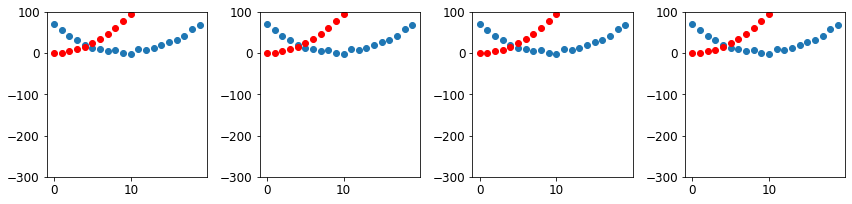## MNIST¶

### Loss Function our 3 and 7 recognizer. Currently we use metric not loss¶

In :
train_x = torch.cat([stacked_threes, stacked_sevens]).view(-1, 28*28)

In :
train_x.size()

Out:
torch.Size([12396, 784])
In :
train_y = tensor(*len(threes) + *len(sevens)).unsqueeze(1)
train_x.shape,train_y.shape

Out:
(torch.Size([12396, 784]), torch.Size([12396, 1]))

### How tensor manipulated¶

In :
temp_tensor = tensor (1)

In :
temp_tensor

Out:
tensor(1)
In :
type(temp_tensor)

Out:
torch.Tensor

is above tensor is wrong what's the difference?

we have a tensor

In :
temp_tensor = tensor()


then we multiuplied the inside of

In :
temp_tensor =tensor(*4)

In :
temp_tensor

Out:
tensor([1, 1, 1, 1])
In :
temp_tensor.shape

Out:
torch.Size()
In :
temp_tensor.ndim

Out:
1
In :
temp_tensor.size()

Out:
torch.Size()
In :
(temp_tensor).unsqueeze(1)

Out:
tensor([,
,
,
])

Warning: looked changed but why size is still unchanged why not [4,1]

In :
temp_tensor.shape

Out:
torch.Size()
In :
temp_tensor.size()

Out:
torch.Size()

### How unsqueeze works?¶

Warning: Whaaaaaaaaaaaaat?

(temp_tensor).unsqueeze(1) doesn't work but (temp_tensor*1).unsqueeze(1) you need to unsqueeze it when creating otherwise it doesnt work. I do not believe it.

In :
temp_tensor = tensor().unsqueeze(1)

In :
temp_tensor.shape

Out:
torch.Size([1, 1])
In :
temp_tensor =tensor(*1).unsqueeze(1)


### Dataset¶

In :
dset = list(zip(train_x,train_y))
x,y = dset
x.shape,x.ndim,y

Out:
(torch.Size(), 1, tensor())

we create list of tuples, each tuple contains a image and a target

In :
valid_x = torch.cat([valid_3_tens, valid_7_tens]).view(-1, 28*28)
valid_y = tensor(*len(valid_3_tens) + *len(valid_7_tens)).unsqueeze(1)
valid_dset = list(zip(valid_x,valid_y))


same for validation

### Weights¶

this is not clear on the videos but consider a layer NN of 728 inputs and 1 output.

In :
def init_params(size, std=1.0): return (torch.randn(size)*std).requires_grad_()

In :
weights = init_params((28*28,1))

In :
weights.shape

Out:
torch.Size([784, 1])
In :
bias = init_params(1)


Note: The function weights*pixels won't be flexible enough—it is always equal to 0 when the pixels are equal to 0 (i.e., its intercept is 0). You might remember from high school math that the formula for a line is y=w*x+b; we still need the b. We'll initialize it to a random number too:

In :
bias

Out:
tensor([0.0959], requires_grad=True)

Again transposing the weight matrix is not clear but Tariq Rashed's book would be very beneficial at this point

In :
(train_x*weights.T).sum() + bias

Out:
tensor([-5.6867], grad_fn=<AddBackward0>)

for all dataset put this multiplication in a function

In :
def linear1(xb): return xb@weights + bias
preds = linear1(train_x)
preds

Out:
tensor([[ -5.6867],
[ -6.5451],
[ -2.0241],
...,
[-14.3286],
[  4.3505],
[-12.6773]], grad_fn=<AddBackward0>)

Create a tensor with results based on their value (above 0.5 is 7 and below it is 3)

In :
corrects = (preds>0.5).float() == train_y
corrects

Out:
tensor([[False],
[False],
[False],
...,
[ True],
[False],
[ True]])

check it

In :
corrects.float().mean().item()

Out:
0.4636172950267792

almost half of them is 3 and the other half is 7 (since weighs are totally random)

### Why we need a loss Function¶

Basically we need to have gradients for correcting our weighs, we need to know which direction we need to go

If you dont understand all of these, ckeck khan academy for gradient.

In :
trgts  = tensor([1,0,1])
prds   = tensor([0.9, 0.4, 0.2])

In :
def mnist_loss(predictions, targets):

In :
torch.where(trgts==1, 1-prds, prds)

Out:
tensor([0.1000, 0.4000, 0.8000])
In :
mnist_loss(prds,trgts)

Out:
tensor(0.4333)

### Sigmoid¶

We need this for squishing predictions between 0-1

In :
def sigmoid(x): return 1/(1+torch.exp(-x))

In :
plot_function(torch.sigmoid, title='Sigmoid', min=-4, max=4)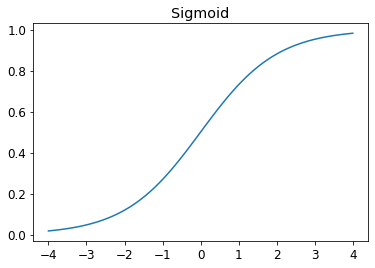update the fuction with the sigmoid thats all.

In :
def mnist_loss(predictions, targets):
predictions = predictions.sigmoid()


### What are SGD and Mini-Batches¶

This explains most of it.

In :
coll = range(15)
list(dl)

Out:
[tensor([ 0,  2, 10, 13,  8]),
tensor([11, 12,  4,  1,  5]),
tensor([ 3, 14,  6,  9,  7])]

but this is only a list however we neeed a tuple consist of independent and dependent variable.

In :
ds = L(enumerate(string.ascii_lowercase))
ds

Out:
(#26) [(0, 'a'),(1, 'b'),(2, 'c'),(3, 'd'),(4, 'e'),(5, 'f'),(6, 'g'),(7, 'h'),(8, 'i'),(9, 'j')...]

then put it into a Dataloader.

In :
dl = DataLoader(ds, batch_size=6, shuffle=True)
list(dl)

Out:
[(tensor([ 1, 23,  9,  8, 24,  2]), ('b', 'x', 'j', 'i', 'y', 'c')),
(tensor([14, 25, 13, 11, 19,  5]), ('o', 'z', 'n', 'l', 't', 'f')),
(tensor([ 0, 10,  4,  7, 18, 12]), ('a', 'k', 'e', 'h', 's', 'm')),
(tensor([ 6, 21, 15, 16, 22,  3]), ('g', 'v', 'p', 'q', 'w', 'd')),
(tensor([20, 17]), ('u', 'r'))]

now we have batches and tuples

all together

It's time to implement the process we saw in <>. In code, our process will be implemented something like this for each epoch:

for x,y in dl:
pred = model(x)
loss = loss_func(pred, y)
loss.backward()

In :
weights = init_params((28*28,1))
bias = init_params(1)

In :
dl = DataLoader(dset, batch_size=256)
xb,yb = first(dl)
xb.shape,yb.shape

Out:
(torch.Size([256, 784]), torch.Size([256, 1]))
In :
valid_dl = DataLoader(valid_dset, batch_size=256)


a small test

In :
batch = train_x[:4]
batch.shape

Out:
torch.Size([4, 784])

predictions

In :
preds = linear1(batch)
preds

Out:
tensor([[ 8.0575],
[14.3841],
[-3.8017],
[ 5.1179]], grad_fn=<AddBackward0>)

loss

In :
loss = mnist_loss(preds, train_y[:4])
loss

Out:
tensor(0.2461, grad_fn=<MeanBackward0>)

In :
loss.backward()

Out:
(torch.Size([784, 1]), tensor(-0.0010), tensor([-0.0069]))

for the step we need a optimizer

put all into a function except the optimizer.

In :
def calc_grad(xb, yb, model):
preds = model(xb)
loss = mnist_loss(preds, yb)
loss.backward()

In :
calc_grad(batch, train_y[:4], linear1)

Out:
(tensor(-0.0021), tensor([-0.0138]))

Warning: if you do it twice results are change.

In :
calc_grad(batch, train_y[:4], linear1)

Out:
(tensor(-0.0031), tensor([-0.0207]))
In :
weights.grad.zero_()

In :
def train_epoch(model, lr, params):
for xb,yb in dl:
for p in params:


little conversion to our results, it's important because we need to understand that what our model says about the numbers(three or not three)

In :
(preds>0.0).float() == train_y[:4]

Out:
tensor([[ True],
[ True],
[False],
[ True]])
In :
def batch_accuracy(xb, yb):
preds = xb.sigmoid()
correct = (preds>0.5) == yb
return correct.float().mean()


this is training accuracy

In :
batch_accuracy(linear1(batch), train_y[:4])

Out:
tensor(0.7500)

this is for validation for all set

In :
def validate_epoch(model):
accs = [batch_accuracy(model(xb), yb) for xb,yb in valid_dl]
return round(torch.stack(accs).mean().item(), 4)

In :
validate_epoch(linear1)

Out:
0.5136

### Training¶

one epochs of training

In :
lr = 1.
params = weights,bias
train_epoch(linear1, lr, params)
validate_epoch(linear1)

Out:
0.7121

then more

In :
for i in range(20):
train_epoch(linear1, lr, params)
print(validate_epoch(linear1), end=' ')

0.8656 0.9203 0.9457 0.9549 0.9593 0.9623 0.9652 0.9666 0.9681 0.9705 0.9706 0.9711 0.972 0.973 0.9735 0.9735 0.974 0.9745 0.9755 0.9755

### Optimizer¶

Let's start creating our model with Pytorch instead of our "linear1" function. Pytorch also creates parameters like our init_params function.

In :
linear_model = nn.Linear(28*28,1)

In :
w,b = linear_model.parameters()

In :
w.shape, b.shape

Out:
(torch.Size([1, 784]), torch.Size())

Custom optimizer

In :
class BasicOptim:
def __init__(self,params,lr): self.params,self.lr = list(params),lr

def step(self, *args, **kwargs):
for p in self.params: p.data -= p.grad.data * self.lr

for p in self.params: p.grad = None

In :
opt = BasicOptim(linear_model.parameters(), lr)


new training fuction will be

In :
def train_epoch(model):
for xb,yb in dl:
opt.step()

In :
validate_epoch(linear_model)

Out:
0.4078
In :
def train_model(model, epochs):
for i in range(epochs):
train_epoch(model)
print(validate_epoch(model), end=' ')

In :
train_model(linear_model, 20)

0.4932 0.8193 0.8418 0.9136 0.9331 0.9477 0.9555 0.9629 0.9658 0.9673 0.9697 0.9717 0.9736 0.9751 0.9761 0.9761 0.9775 0.9775 0.9785 0.9785

### Fastai's SDG class¶

instead of using "BasicOptim" class we can use fastai's SGD class

In :
linear_model = nn.Linear(28*28,1)
opt = SGD(linear_model.parameters(), lr)
train_model(linear_model, 20)

0.4932 0.7808 0.8623 0.9185 0.9365 0.9521 0.9575 0.9638 0.9658 0.9678 0.9707 0.9726 0.9741 0.9751 0.9761 0.9765 0.9775 0.978 0.9785 0.9785

Just remove the "train_model" at this time and use fastai's "Learner.fit" Before using Learner first we need to pass our trainig and validation data into "Dataloaders" not "dataloader"

In :
dls = DataLoaders(dl, valid_dl)

In :
learn = Learner(dls, nn.Linear(28*28,1), opt_func=SGD,
loss_func=mnist_loss, metrics=batch_accuracy)


#### FastAi's Fit¶

In :
learn.fit(10, lr=lr)

epoch train_loss valid_loss batch_accuracy time
0 0.637166 0.503575 0.495584 00:00
1 0.562232 0.139727 0.900393 00:00
2 0.204552 0.207935 0.806183 00:00
3 0.088904 0.114767 0.904809 00:00
4 0.046327 0.081602 0.930324 00:00
5 0.029754 0.064530 0.944553 00:00
6 0.022963 0.054135 0.954858 00:00
7 0.019966 0.047293 0.961236 00:00
8 0.018464 0.042515 0.965162 00:00
9 0.017573 0.039011 0.966634 00:00

The basic idea is that by using more linear layers, we can have our model do more computation, and therefore model more complex functions. But there's no point just putting one linear layer directly after another one, because when we multiply things together and then add them up multiple times, that could be replaced by multiplying different things together and adding them up just once! That is to say, a series of any number of linear layers in a row can be replaced with a single linear layer with a different set of parameters. (From Fastbook)

Amazingly enough, it can be mathematically proven that this little function can solve any computable problem to an arbitrarily high level of accuracy, if you can find the right parameters for w1 and w2 and if you make these matrices big enough. For any arbitrarily wiggly function, we can approximate it as a bunch of lines joined together; to make it closer to the wiggly function, we just have to use shorter lines. This is known as the universal approximation theorem._ The three lines of code that we have here are known as layers. The first and third are known as linear layers, and the second line of code is known variously as a nonlinearity, or activation function.(From Fastbook)

In :
simple_net = nn.Sequential(
nn.Linear(28*28,30),
nn.ReLU(),
nn.Linear(30,1)
)

In :
learn = Learner(dls, simple_net, opt_func=SGD,
loss_func=mnist_loss, metrics=batch_accuracy)

In :
learn.fit(40, 0.1)

epoch train_loss valid_loss batch_accuracy time
0 0.303284 0.398378 0.511776 00:00
1 0.142384 0.221517 0.817959 00:00
2 0.079702 0.112610 0.917076 00:00
3 0.052855 0.076474 0.942100 00:00
4 0.040301 0.059791 0.958783 00:00
5 0.033824 0.050389 0.964181 00:00
6 0.030075 0.044483 0.966143 00:00
7 0.027629 0.040465 0.966634 00:00
8 0.025865 0.037553 0.969578 00:00
9 0.024499 0.035336 0.971541 00:00
10 0.023391 0.033579 0.972522 00:00
11 0.022467 0.032142 0.973994 00:00
12 0.021679 0.030936 0.973994 00:00
13 0.020997 0.029901 0.974975 00:00
14 0.020398 0.028998 0.974975 00:00
15 0.019869 0.028198 0.975957 00:00
16 0.019395 0.027484 0.976448 00:00
17 0.018966 0.026841 0.976938 00:00
18 0.018577 0.026259 0.977429 00:00
19 0.018220 0.025730 0.977429 00:00
20 0.017892 0.025244 0.978410 00:00
21 0.017588 0.024799 0.979882 00:00
22 0.017306 0.024388 0.979882 00:00
23 0.017042 0.024008 0.980373 00:00
24 0.016794 0.023656 0.980864 00:00
25 0.016561 0.023328 0.980864 00:00
26 0.016341 0.023022 0.980864 00:00
27 0.016133 0.022737 0.981845 00:00
28 0.015935 0.022470 0.981845 00:00
29 0.015746 0.022221 0.981845 00:00
30 0.015566 0.021988 0.982336 00:00
31 0.015395 0.021769 0.982336 00:00
32 0.015231 0.021565 0.982826 00:00
33 0.015076 0.021371 0.982826 00:00
34 0.014925 0.021190 0.982826 00:00
35 0.014782 0.021018 0.982826 00:00
36 0.014643 0.020856 0.982826 00:00
37 0.014510 0.020703 0.982826 00:00
38 0.014382 0.020558 0.982826 00:00
39 0.014258 0.020420 0.982826 00:00

#### recorder is a fast ai method¶

In :
plt.plot(L(learn.recorder.values).itemgot(2));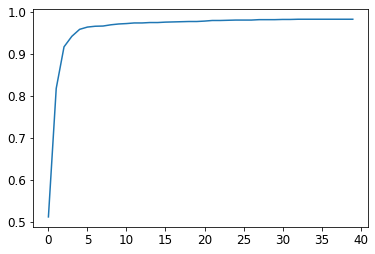Last value

In :
learn.recorder.values[-1]

Out:
0.982826292514801

## GOING DEEPER¶

why deeper if it is two and a nonlinear between them is enough

We already know that a single nonlinearity with two linear layers is enough to approximate any function. So why would we use deeper models? The reason is performance. With a deeper model (that is, one with more layers) we do not need to use as many parameters; it turns out that we can use smaller matrices with more layers, and get better results than we would get with larger matrices, and few layers.

In :
dls = ImageDataLoaders.from_folder(path)
learn = cnn_learner(dls, resnet18, pretrained=False,
loss_func=F.cross_entropy, metrics=accuracy)
learn.fit_one_cycle(1, 0.1)

epoch train_loss valid_loss accuracy time
0 0.089727 0.011755 0.997056 00:13
In [ ]: